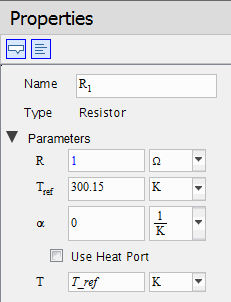Cross Connect Thermal Conductor - MapleSim Help

Cross Connect Thermal Conductor

Cross Connect Thermal Conductor connecting two Node or Shape components with contact area of each node, input from real vector signals.Description The Cross Connect Thermal Conductor component is used for connecting two Node or Shape components with all combinations of heat path. This component can also take account of normalized contact area ratio between two Node or node of the Shape from two real vector signals, and $\mathrm{Ac_b}\left[i\right]$. The order of coefficients of normalized contact area ratio and $\mathrm{Ac_b}$ are as follows: For example, when  and , $\mathrm{Ac_b}\left[4\right]$ means $\mathrm{a}\left[2\right]-\mathrm{b}\left[1\right],$the coefficient of normalized area ratio of $\mathrm{port_b}$ side between and $\mathrm{port_b}\left[1\right]$. When using this component, connect and to of Node or Shape components, and input two real vector signals of coefficient of normalized contact area ratio (0 to 1).The following image illustrates the behavior of the Cross Connect Thermal Conductor component in the case of $\mathrm{numNode_a}$=2 and $\mathrm{numNode_b}$=3. There are $2×3=6$Equations Heat conductance $\mathrm{G__off}$ is used for the heat path without heat flow. (For details, see Thermal Conductor help).Variables (For details, see Thermal Conductor  help).Connections

 Name Description Modelica ID $\mathrm{port_a}\left[i\right]$ i-th thermal port of port a side The total number of i is determined by Nodes of a port_a $\mathrm{port_b}\left[i\right]$ i-th thermal port of port b side The total number of i is determined by Nodes of b port_b $\mathrm{Ac_a}\left[i\right]$ Coefficient of normalized contact area ratio (0 to1) at port a side The total number of i is determined by Nodes a * Nodes b Ac_a $\mathrm{Ac_b}\left[i\right]$ Coefficient of normalized contact area ratio (0 to 1) at port b side The total number of i is determined by Nodes a * Nodes b Ac_bParameters

 Symbol Default Units Description Modelica ID $\mathrm{Material__a}$ $\mathrm{SolidPropertyData1}$ $-$ Solid material property data Material_a $\frac{W}{m\cdot K}$ Material.k is the thermal conductivity of the material Material_a.k $\frac{J}{\mathrm{kg}\cdot K}$ Material.cp is the specific heat capacity of the material Material_a.cp $\frac{\mathrm{kg}}{{m}^{3}}$ Material.rho is the density of the material Material_a.rho $\mathrm{Material__b}$ $\mathrm{SolidPropertyData1}$ $-$ Solid material property data Material_b $\frac{W}{m\cdot K}$ Material.k is the thermal conductivity of the material Material_b.k $\frac{J}{\mathrm{kg}\cdot K}$ Material.cp is the specific heat capacity of the material Material_b.cp $\frac{\mathrm{kg}}{{m}^{3}}$ Material.rho is the density of the material Material_b.rho $\mathrm{Nodes__a}$ 2 Number of nodes port a side $\mathrm{numNode_a}$ $\mathrm{Nodes__b}$ 3 Number of nodes port b side $\mathrm{numNode_b}$ $\mathrm{A__port a}$ $1$ ${m}^{2}$ Area of Thermal Conductor for each node of port a side A_a $\mathrm{L__port a}$ $1$ ${m}^{}$ Distance of Thermal Conductor for each node of port a side L_a $\mathrm{A__port b}$ $1$ ${m}^{2}$ Area of Thermal Conductor for each node of port b side A_b $\mathrm{L__port b}$ $1$ ${m}^{}$ Distance of Thermal Conductor for each node of port b side L_b $\mathrm{G__off}$ ${10}^{-7}$ $\frac{W}{K}$ Optional. Constant thermal conductance of material which is used for representing the path without heat flow GoffSee Also